# Moore space

(diff) ← Older revision | Latest revision (diff) | Newer revision → (diff)

A topological spacewith a unique non-trivial reduced homology group: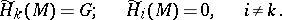Ifis the Eilenberg–MacLane space of the group of integers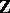and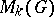is the Moore space with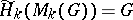, then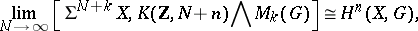that is,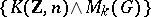is the spectrum of the cohomology theory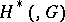. This allows one to extend the idea of cohomology with arbitrary coefficients to a generalized cohomology theory. For any spectrum, the spectrumdefines a cohomology theory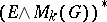, called the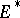-cohomology theory with coefficient group. For the definition of generalized homology theories with coefficients in a group, the so-called co-Moore spaceis used, which is characterized by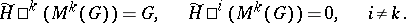For example, the group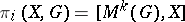is called the homotopy group of the spacewith coefficients in. However, the space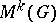does not exist for all pairs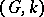. Ifis a finitely-generated group, thendoes exist.

How to Cite This Entry:
Moore space. Encyclopedia of Mathematics. URL: http://encyclopediaofmath.org/index.php?title=Moore_space&oldid=18047
This article was adapted from an original article by A.F. Kharshiladze (originator), which appeared in Encyclopedia of Mathematics - ISBN 1402006098. See original article